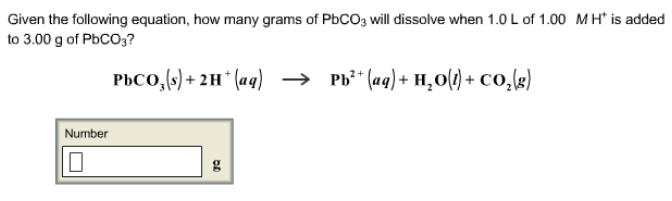# Problem: Given the following equation, how many grams of PbCO3 will dissolve when 1.0 L of 1.00 M H+ is added to 3.00 g of PbCO3? PbCO3 (s) + 2H+ (aq) → Pb2+ (aq) + H2O (l) + CO2 (s)

###### FREE Expert Solution
95% (136 ratings)###### Problem Details

Given the following equation, how many grams of PbCO3 will dissolve when 1.0 L of 1.00 M H+ is added to 3.00 g of PbCO3

PbCO3 (s) + 2H+ (aq) → Pb2+ (aq) + H2O (l) + CO2 (s)